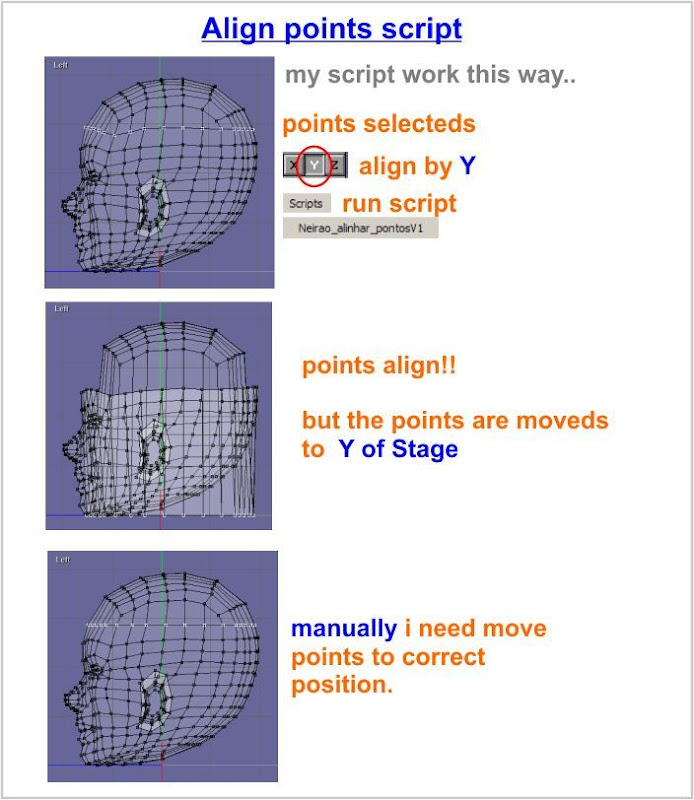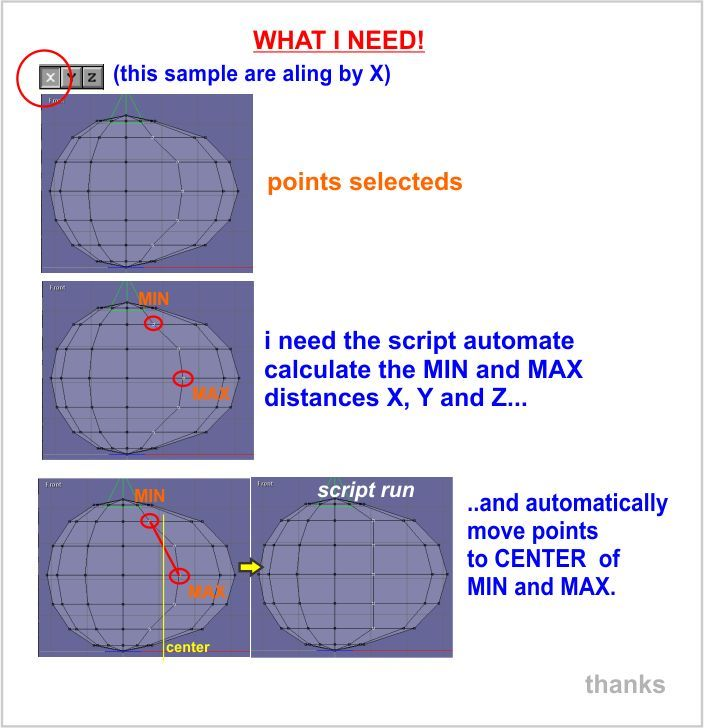# Anim8or Community

• January 31, 2023, 02:15:11 am• Welcome, Guest### News:

Ian Ross has just released a book on Anim8or. It's perect for a beginner and a good reference for experienced users. It contains detailed chapters on every aspect, with many examples. Get your own copy here: "Anim8or Tutorial Book"

Pages: 

###AuthorTopic: ALIGN POINTS SCRIPT - Help need  (Read 6706 times)

#### neirao

• Sr. Member
•• Posts: 622
•• Neirao##### ALIGN POINTS SCRIPT - Help need
« on: May 13, 2011, 01:39:48 pm »

Hi Guys,

so i have this script for many time,
its work for me, but i think then can be better.i show example:i need..here the script:
Code: [Select]
`/*  *Name: Aling points to (x,y,z)  *CopyLeft: 2010  *Author: Neirão  *Date: 03/03/10  *Description: based in Vytautas_alinhar_pontos_line_points_v1   *Parameters:  *= width;  *= height;  *= width_divisions;  *= height_divisions;  *//***** Init *****/#command("object");object \$object;shape \$shapes;shape \$shape;int \$shape_count;point3 \$points;point3 \$point;int \$point_count;int \$i;int \$j;int \$direction;if (GUI.Xenabled) \$direction = 0;else if (GUI.Yenabled) \$direction = 1;else \$direction = 2;if (GUI.Xenabled && GUI.Yenabled && GUI.Zenabled) \$direction = 3;meshdata \$mesh_data; \$object = project.curObject;\$shape_count = \$object.GetShapes(\$shapes);/*** Get all selected points from all the shapes ***/for \$i = 0 to \$shape_count - 1 do{ \$shape = \$shapes[\$i]; /* If shape is a mesh.. */ if(\$shape.GetKind() == SHAPE_KIND_MESH){/* i try this..but dont work.. :( --> || \$shape.GetKind() == SHAPE_KIND_SUBDIVISION */ /* Get the shape point number */ \$point_count = \$shape.GetNumPoints(); for \$j = 0 to \$point_count - 1 do{ if(\$shape.GetPointSelected(\$j)) { \$point = \$shape.GetPoint(\$j); if(\$direction == 0){ \$point.x = 0; } if(\$direction == 1){ \$point.y = 0; } if(\$direction == 2){ \$point.z = 0; } /* TODOS XYZ ATIVADOS alinha todos para  */ if(\$direction == 3){ \$point.x = 0; \$point.y = 0; \$point.z = 0; } \$shape.SetPoint(\$j, \$point); } } }} `
can someone maker this correction??!thanks !Logged

#### NickE

• Full Member
•• Posts: 162
•##### Re: ALIGN POINTS SCRIPT - Help need
« Reply #1 on: May 13, 2011, 04:41:53 pm »

Neirao,
Below is your code with the changes you requested:
Code: [Select]
`/*  *Name: Aling points to (x,y,z)  *CopyLeft: 2010  *Author: Neirão  *Date: 03/03/10  *Description: based in Vytautas_alinhar_pontos_line_points_v1   *Parameters:  *= width;  *= height;  *= width_divisions;  *= height_divisions;  *//***** Init *****/#command("object");object \$object;shape \$shapes;shape \$shape;int \$shape_count;point3 \$points;point3 \$point;int \$point_count;int \$i;int \$j;int \$direction;/* added by NickE */point3 \$sel_max,\$sel_min,\$sel_center;/* end of added by NickE */if (GUI.Xenabled) \$direction = 0;else if (GUI.Yenabled) \$direction = 1;else \$direction = 2;if (GUI.Xenabled && GUI.Yenabled && GUI.Zenabled) \$direction = 3;meshdata \$mesh_data; \$object = project.curObject;\$shape_count = \$object.GetShapes(\$shapes);/*** Get all selected points from all the shapes ***/for \$i = 0 to \$shape_count - 1 do{ \$shape = \$shapes[\$i]; /* If shape is a mesh.. */ if(\$shape.GetKind() == SHAPE_KIND_MESH){/* i try this..but dont work.. :( --> || \$shape.GetKind() == SHAPE_KIND_SUBDIVISION */ /* Get the shape point number */ \$point_count = \$shape.GetNumPoints(); /* added by NickE */        \$sel_max=(-10000,-10000,-10000);        \$sel_min=( 10000, 10000, 10000);        for \$j = 0 to \$point_count - 1 do        {           if (\$shape.GetPointSelected(\$j))           {              \$point = \$shape.GetPoint(\$j);              if (\$point.x > \$sel_max.x) \$sel_max.x = \$point.x;              if (\$point.y > \$sel_max.y) \$sel_max.y = \$point.y;              if (\$point.z > \$sel_max.z) \$sel_max.z = \$point.z;              if (\$point.x < \$sel_min.x) \$sel_min.x = \$point.x;              if (\$point.y < \$sel_min.y) \$sel_min.y = \$point.y;              if (\$point.z < \$sel_min.z) \$sel_min.z = \$point.z;           }        }        \$sel_center.x=(\$sel_max.x + \$sel_min.x)/2.0;                \$sel_center.y=(\$sel_max.y + \$sel_min.y)/2.0;                \$sel_center.z=(\$sel_max.z + \$sel_min.z)/2.0; /* End of added by Nick E */         for \$j = 0 to \$point_count - 1 do{ if(\$shape.GetPointSelected(\$j)) { \$point = \$shape.GetPoint(\$j); if(\$direction == 0){ /* \$point.x = 0; */                        \$point.x = \$sel_center.x;   /* Added by NickE */ } if(\$direction == 1){ /* \$point.y = 0; */                        \$point.y = \$sel_center.y;   /* Added by NickE */ } if(\$direction == 2){ /* \$point.z = 0; */                        \$point.z = \$sel_center.z;   /* Added by NickE */ } /* TODOS XYZ ATIVADOS alinha todos para  */ if(\$direction == 3){ \$point.x = 0; \$point.y = 0; \$point.z = 0; } \$shape.SetPoint(\$j, \$point); } } }} `
1) Do you really want the center, or do you really want the average?
2) This script works on the actual coordinates of the points, so if a mesh is moved or rotated, it still operating on the underlying point coordinates rather than the transformed coordinates.  This may give different results than what is expected.Logged

#### neirao

• Sr. Member
•• Posts: 622
•• Neirao##### Re: ALIGN POINTS SCRIPT - Help need
« Reply #2 on: May 13, 2011, 05:54:02 pm »

lolthanks Nicke! you are very good in a8s!

at first i wanted in "really center" but no is necessary
but this way are good!
your modifiers is very usefull for me!

thanks so much!Logged
Pages: